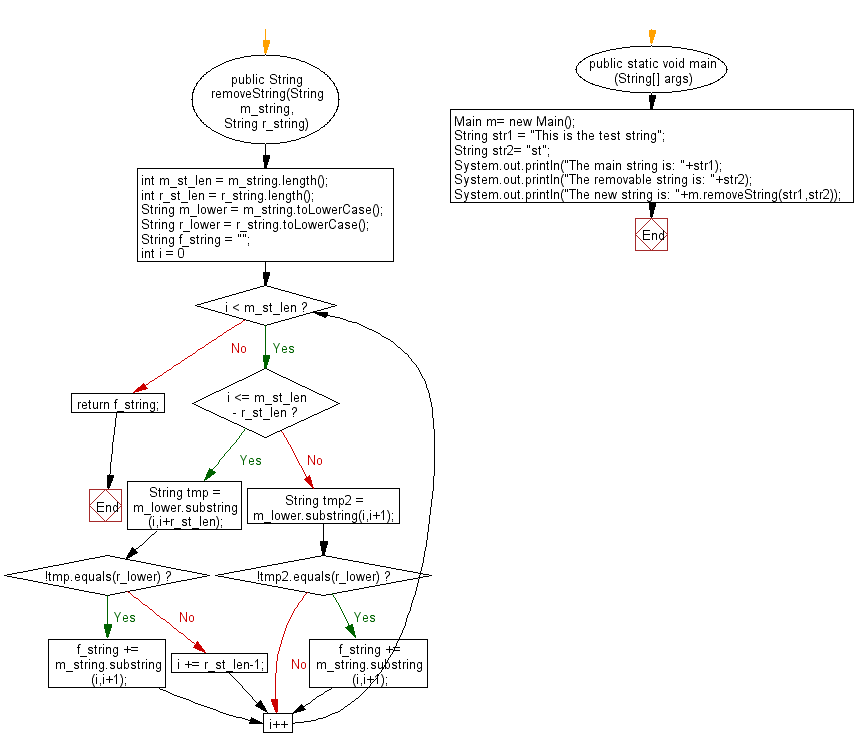﻿ Java exercises: Return a substring after removing the all instances of remove string as given from the given main string - w3resource# Java String Exercises: Return a substring after removing the all instances of remove string as given from the given main string

## Java String: Exercise-92 with Solution

Write a Java program to return a substring after removing the all instances of remove string as given from the given main string.

Sample Solution:

Java Code:

``````import java.util.*;
public class Main
{
public String removeString(String m_string, String r_string)
{
int m_st_len = m_string.length();
int r_st_len = r_string.length();
String m_lower  = m_string.toLowerCase();
String r_lower  = r_string.toLowerCase();
String f_string = "";
for (int i = 0; i < m_st_len; i++)
{
if (i <= m_st_len - r_st_len)
{
String tmp = m_lower.substring(i,i+r_st_len);
if (!tmp.equals(r_lower))
f_string += m_string.substring(i,i+1);
else
{
i += r_st_len-1;
}
}
else
{
String tmp2 = m_lower.substring(i,i+1);
if (!tmp2.equals(r_lower))
f_string += m_string.substring(i,i+1);
}
}
return f_string;
}

public static void main (String[] args)
{
Main m= new Main();
String str1 =  "This is the test string";
String str2= "st";
System.out.println("The main string is: "+str1);
System.out.println("The removable string is: "+str2);
System.out.println("The new string is: "+m.removeString(str1,str2));
}
}
``````

Sample Output:

```The main string is: This is the test string
The removable string is: st
The new string is: This is the te ring
```

Pictorial Presentation:Flowchart:Java Code Editor:

Improve this sample solution and post your code through Disqus

What is the difficulty level of this exercise?

﻿

## Java: Tips of the Day

Array vs ArrayLists:

The main difference between these two is that an Array is of fixed size so once you have created an Array you cannot change it but the ArrayList is not of fixed size. You can create instances of ArrayLists without specifying its size. So if you create such instances of an ArrayList without specifying its size Java will create an instance of an ArrayList of default size.

Once an ArrayList is full it re-sizes itself. In fact, an ArrayList is internally supported by an array. So when an ArrayList is resized it will slow down its performance a bit as the contents of the old Array must be copied to a new Array.

At the same time, it's compulsory to specify the size of an Array directly or indirectly while creating it. And also Arrays can store both primitives and objects while ArrayLists only can store objects.

Ref: https://bit.ly/3o8L2KH